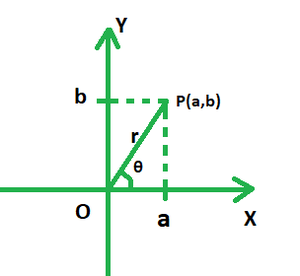# Find the distance using the modulus of the difference between z1 = -8 + 3i and z2 = 4 + 7i

• Last Updated : 01 Jan, 2022

A complex number can be called a summation of both real and imaginary numbers written or represented usually in the form of z = a + ib, where i (iota) is the imaginary component and represents √(-1). Complex numbers are commonly depicted in their rectangular or standard form as a + ib. For example, 69 + 25i is a complex number where 100 is the real part and 25i is the imaginary part.

Complex numbers can be purely real or purely imaginary depending upon the values of either of each of the two components.

ModulusWhen a complex number is presented on a graph, its real part is plotted on the x-axis and the imaginary part on the y-axis. Say if the number were to be represented by point P in the figure given below, triangles OPA and OPB would be both right-angled. Clearly, in the right triangle POA, PO is the hypotenuse; OA is the base and PA is the perpendicular. Using Pythagoras Theorem, we have:

OP2 = OA2 + PA2

OP =The absolute value of a complex number is regarded as its modulus. It is the square root of the sum of squares of its real and imaginary parts. In the above case, OP is the modulus of the complex number of the form z = a + ib, and is denoted by r.

### Find the distance using the modulus of the difference between z1 = -8 + 3i and z2 = 4 + 7i.

Solution:

Difference between the given complex numbers:

z1 – z2 = −8 + 3i – (4 + 7i)

= −8 – 4 + 3i – 7i

z = –12 – 4i

Distance between z1 and z2 = Modulus of z =units

### Similar Problems

Question 1. Find the distance using the modulus of the difference between z1 = 12 + 3i and z2 = 10 – 5i.

Solution:

Difference between the given complex numbers:

z1 – z2 = 12 + 3i – (10 – 5i)

= 12 – 10 + 3i – 5i

z = 2 – 2i

Distance between z1 and z2 = Modulus of z =units

Question 2. Find the distance using the modulus of the difference between z_1 = 5 + 3i and z_2 = 4 + 7i.

Solution:

Difference between the given complex numbers:

z1 – z2 = 5 + 3i – (4+7i)

= 5 – 4 + 3i – 7i

z = 1 – 4i

Distance between z1 and z2 = Modulus of z =units

Question 3. Find the distance using the modulus of the difference between z_1 = 9 + 3i and z_2 = 4 + 5i.

Solution:

Difference between the given complex numbers:

z1 – z2 = 9 + 3i – (4 + 5i)

= 9 – 4 + 3i – 5i

z = 5 – 2i

Distance between z1 and z2 = Modulus of z =units

Question 4. Find the distance using the modulus of the difference between z_1 = 6 + 3i and z_2 = 4 + 7i.

Solution:

Difference between the given complex numbers:

z1 – z2 = 6 + 3i – (4 + 7i)

= 6 – 4 + 3i – 7i

z = 2 – 4i

Distance between z1 and z2 = Modulus of z =units

Question 5. Find the distance using the modulus of the difference between z_1 = 69 + 3i and z_2 = 68 + 7i.

Solution:

Difference between the given complex numbers:

z1 – z2 = 69 + 3i – (68 + 7i)

= 69 – 68 + 3i – 7i

z = 1 – 4i

Distance between z1 and z2 = Modulus of z =units

My Personal Notes arrow_drop_up